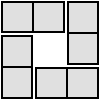#### You may also like### 4 Dom

Use these four dominoes to make a square that has the same number of dots on each side.### Pair Sums

Five numbers added together in pairs produce: 0, 2, 4, 4, 6, 8, 9, 11, 13, 15 What are the five numbers?### Number Sandwiches

Can you arrange the digits 1, 1, 2, 2, 3 and 3 to make a Number Sandwich?

# One to Eight

##### Age 11 to 14Challenge Level

Sue from CBEC Basingstoke sent the following explanation to the first question:

** X ** = 4876
If you write 4876 as a product of prime factors you get 2 x 2 x 23 x 53
From this you need to find two, two digit numbers that use the digits 2, 3, 5 and 9
This has to be 2 x 2 x 23 = 92 and 53

We also received correct solutions from Jenny, Greenian, Rachael, Jacqui and Sam, from The Mount School, and Debbie from Forres Academy. Well done to you all.

53 x 92 = 4876
62 x 87 = 5394

24 x 57 = 1368
58 x 64 = 3712
52 x 34 = 1768

We'd like to hear from anyone who would like to explain how they solved these problems.
We are always interested in the reasoning that has helped you reach your solutions.